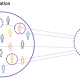###### Monte Carlo Simulation Explained
2021-10-072021-10-08

# Overview

Inverse transform sampling is a method for generating random numbers from any probability distribution by using its inverse cumulative distribution F−1(x)F−1(x). Recall that the cumulative distribution for a random variable XX is FX(x)=P(X≤x)FX(x)=P(X≤x). In what follows, we assume that our computer can, on demand, generate independent realizations of a random variable UU uniformly distributed on [0,1][0,1].

# Algorithm

## Continuous Distributions

Assume we want to generate a random variable XX with cumulative distribution function (CDF) FXFX. The inverse transform sampling algorithm is simple:
1. Generate U∼Unif(0,1)U∼Unif(0,1)
2. Let X=F−1X(U)X=FX−1(U).

Then, XX will follow the distribution governed by the CDF FXFX, which was our desired result.

Note that this algorithm works in general but is not always practical. For example, inverting FXFX is easy if XX is an exponential random variable, but its harder if XX is Normal random variable.

## Discrete Distributions

Now we will consider the discrete version of the inverse transform method. Assume that XX is a discrete random variable such that P(X=xi)=piP(X=xi)=pi. The algorithm proceeds as follows:
1. Generate U∼Unif(0,1)U∼Unif(0,1)
2. Determine the index kk such that ∑k−1j=1pj≤U<∑kj=1pj∑j=1k−1pj≤U<∑j=1kpj, and return X=xkX=xk.

Notice that the second step requires a search.

Assume our random variable XX can take on any one of KK values with probabilities {p1,…,pK}{p1,…,pK}. We implement the algorithm below, assuming these probabilities are stored in a vector called `p.vec.`

```# note: this inefficient implementation is for pedagogical purposes
# in general, consider using the rmultinom() function
discrete.inv.transform.sample <- function( p.vec ) {
U  <- runif(1)
if(U <= p.vec){
return(1)
}
for(state in 2:length(p.vec)) {
if(sum(p.vec[1:(state-1)]) < U && U <= sum(p.vec[1:state]) ) {
return(state)
}
}
}
```

# Continuous Example: Exponential Distribution

Assume YY is an exponential random variable with rate parameter λ=2λ=2. Recall that the probability density function is p(y)=2e−2yp(y)=2e−2y, for y>0y>0. First, we compute the CDF:FY(x)=P(Y≤x)=∫x02e−2ydy=1−e−2xFY(x)=P(Y≤x)=∫0x2e−2ydy=1−e−2x

Solving for the inverse CDF, we get thatF−1Y(y)=−ln(1−y)2FY−1(y)=−ln⁡(1−y)2

Using our algorithm above, we first generate U∼Unif(0,1)U∼Unif(0,1), then set X=F−1Y(U)=−ln(1−U)2X=FY−1(U)=−ln⁡(1−U)2. We do this in the R code below and compare the histogram of our samples with the true density of YY.

```# inverse transform sampling
num.samples <-  1000
U           <-  runif(num.samples)
X           <- -log(1-U)/2

# plot
hist(X, freq=F, xlab='X', main='Generating Exponential R.V.')
curve(dexp(x, rate=2) , 0, 3, lwd=2, xlab = "", ylab = "", add = T)
```

Past versions of unnamed-chunk-2-1.png

Indeed, the plot indicates that our random variables are following the intended distribution.

# Discrete Example

Let’s assume we want to simulate a discrete random variable XX that follows the following distribution:

Below we simulate from this distribution using the discrete.inv.transform.sample() function above, and plot both the true probability vector, and the empirical proportions from our simulation.

```num.samples <- 1000
p.vec        <- c(0.1, 0.4, 0.2, 0.3)
names(p.vec) <- 1:4
samples     <- numeric(num.samples)
for(i in seq_len(num.samples) ) {
samples[i] <- discrete.inv.transform.sample(p.vec)
}
barplot(p.vec, main='True Probability Mass Function')
```

Past versions of unnamed-chunk-3-1.png

```barplot(table(samples), main='Empirical Probability Mass Function')
```

References:

https://stephens999.github.io/fiveMinuteStats/inverse_transform_sampling.html

https://stats.stackexchange.com/questions/184325/how-does-the-inverse-transform-method-work/184337#184337

https://towardsdatascience.com/an-insight-on-generating-samples-from-a-custom-probability-density-function-d0a06c290c54

https://support.minitab.com/en-us/minitab-express/1/help-and-how-to/basic-statistics/probability-distributions/supporting-topics/basics/using-the-inverse-cumulative-distribution-function-icdf/##### Amir Masoud Sefidian
Data Scientist, Machine Learning Engineer, Researcher, Software Developer